Velocity and Acceleration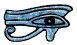# Velocity and Acceleration

Some people find it difficult to understand how something can be accelerating if it isn't even moving. This Page tries to explain how this can be and also some other ideas about velocity and acceleration.

If a body is not moving its distance from any fixed point is constant. We often call the distance of a body from a fixed point its displacement. We measure displacement in metres (m). If we plot a graph of displacement against time for a stationary body we have a graph like this.If the body is moving away from the point at a steady rate in a straight line we say it is moving at a constant velocity. We measure velocity in metres per second (m/s). For a body moving with constant velocity the graphs of velocity and displacement against time look like this.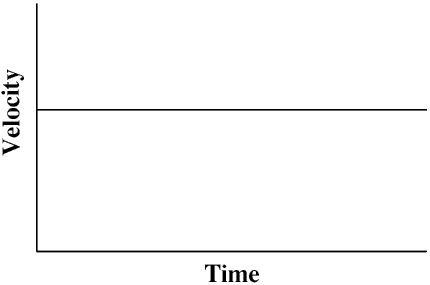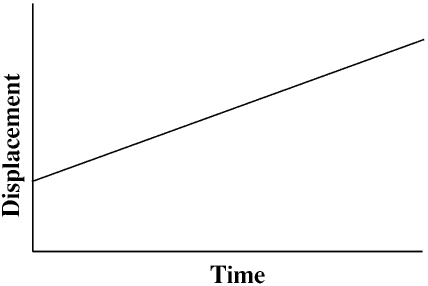If the velocity of the body is changing we say it is accelerating. If a body is accelerating at a constant rate and at one moment is moving at ten metres per second and a second later is moving at twelve metres per second, its velocity is changing at a rate of two metres per second pause per second - it is easier to understand this if you put in a pause as you say it - so its acceleration is 2 m/s2. We can say this as two metres per second per second or two metres per second squared but not as two metres per square second!

For a body accelerating at a constant rate the graphs of acceleration, velocity and displacement against time look like this.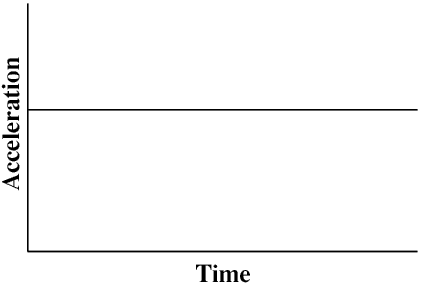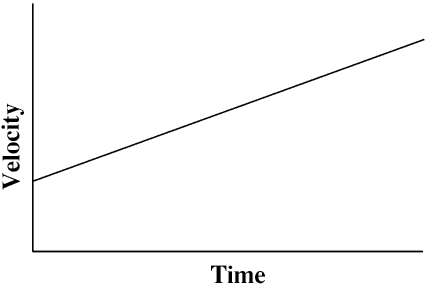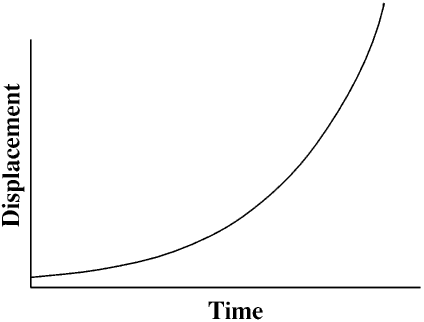To understand what is happening to a body when the acceleration is not constant it may be helpful to consider the shape of the above graphs for when it is constant.

The velocity of a body is the rate at which the displacement (its position) is changing, that is, it is the slope of the displacement/time graph. If the body is not moving the velocity is zero and the displacement/time graph is a horizontal straight line. If the body is moving at a constant velocity the displacement/time graph is a sloping straight line, the higher the velocity the steeper the slope. If the body is accelerating its velocity is changing and so the displacement/time graph will be a curve. But the slope of the curve at any point in time will still be the velocity at that point.

Similarly, the acceleration is the rate of change of velocity, so if the acceleration is zero the velocity/time graph will be a horizontal straight line, if the acceleration is constant it will be a sloping straight line, and if the acceleration is changing it will be a curve, with the slope at any point being the acceleration - an example of this is shown later on this page, in the section on Simple Harmonic Motion.

Remember that displacement, velocity and acceleration are vector quantities, that is, they have both magnitude and direction. If a body is moving in a circle at a constant speed the direction it is moving in is constantly changing and so although its speed is constant its velocity is not. If its velocity is changing it must be accelerating: this acceleration is the cause of the g force when a racing car goes round a corner or an aircraft pulls out of a dive and is why a white-knuckle ride at a fairground can loop the loop, and is discussed on another Page (actually on the tides) on my web site.

Finding the velocity and acceleration given the displacement/time graph involves a branch of mathematics known as the differential calculus. Finding the velocity and displacement given the acceleration/time graph involves the integral calculus and is much more difficult, but much more useful. But these are outside the scope of this Web Page.

For a body moving with a constant acceleration the following formulae can be used.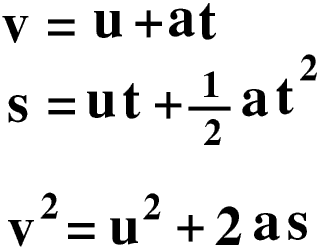t is the time interval s is the distance moved during this time u is the velocity at the start v is the velocity at the end a is the (constant) acceleration

## The acceleration due to gravity

The Earth's gravity is a force which pulls bodies towards it. The gravitational attraction of the Earth varies with the distance from its surface and also from place to place on it, but very close to its surface the Earth's gravitational force acting on a freely falling body produces an acceleration of about 9.81 m/s2. This is called the acceleration due to gravity and is given the symbol g. For most purposes we usually take g to be 9.81 m/s2 but remember that g is not a constant but varies slightly from place to place on the Earth's surface and also with the height above it.

When using the above formulae for calculations involving freely falling bodies the initial velocity (u) is usually zero and the acceleration (a) is usually taken to be 9.81 m/s2.

## Terminal Velocity

If we put our hand out of the window of a stationary car we do not feel any air resistance but if the car is moving we do - the faster the car is moving the greater the air resistance.

The air resistance on a body depends upon its size and shape and its speed. It also depends upon the density of the air - this is why airliners fly very high, where the air density and so air resistance is much less.

If we drop an object off a tall tower, at the moment we release it it has no velocity and so no air resistance. The only force acting on it is its weight and so its acceleration is about 9.81 m/s2. However as it falls its velocity increases and so does the air resistance on it. This force acts in the opposite direction to its weight and will progressively reduce its acceleration. At some point it will reach a velocity at which the force of the air resistance on it is equal to its weight and then the acceleration will be zero and it will not get any faster. This is its terminal velocity. The terminal velocity of a body depends upon its weight and its size and shape (and the density of the air): the terminal velocity of a feather is less than 1 m/s and will be reached by the time the feather has fallen a few centimetres whereas that of a cannon ball is several hundred m/s, and will probably not be reached unless it is dropped out of an aeroplane.

You can do some simple experiments on terminal velocity using empty (preferably unused) fairy cake cases - these reach their terminal velocity within a few centimetres of being released and fall straight down slowly enough to be timed using an ordinary stop watch.

The terminal velocity of an adult jumping out of an aeroplane without any special training or equipment (except a parachute of course!) is about 50 m/s (about 180 km/hour) although a specially equipped and trained sky-diver can reach much higher speeds. When the parachute opens it produces very much more air resistance and so reduces the terminal velocity to less than 10 m/s - but you still hit the ground at a speed equivalent to jumping off a 3 m wall, so unless you have been taught how to land properly you may still injure yourself.

## Simple Harmonic Motion

We need a force to stretch (extend) a spring: the bigger the force the greater the extension. Of course, if we use too big a force we will break the spring.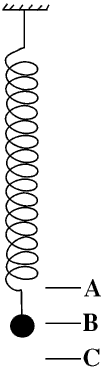If we hang a steel ball from a spring the spring will stretch until the force in it is equal to the weight of the ball - call this point B.

If we then pull down the spring a little more, to point C, the force in the spring will be greater than the weight of the ball and so when we release it it will start to accelerate upwards.

As it moves upwards the force in the spring will get less and so although the ball will still be getting faster the acceleration (that is, the rate at which it is getting faster) will be getting less. By the time it reaches point B the force in the spring will be equal to the weight of the ball so there will be no more acceleration - but the ball will have been accelerating until then so it will be travelling upwards at its maximum velocity.

It continues to move upwards, but now the force in the spring starts to become less than the weight of the ball and the acceleration becomes negative (downwards). The ball slows down and by the time it reaches point A it will have zero velocity - but the force in the spring will be less than the weight of the ball and therefore the acceleration will not be zero. So the ball starts to move down again, and the whole cycle repeats itself. This is called Simple Harmonic Motion (SHM)

Here are the graphs for SHMRemember that the acceleration is always in the opposite direction to the displacement and that the velocity is at its maximum (or minimum) when the acceleration and displacement are zero, and visa versa.

You can check the graphs to see that when the velocity is zero (Points A and C) the gradient of the displacement/time graph is zero, and when the acceleration is zero (Point B) the gradient of the velocity/time graph is zero.

If there is no friction or air resistance etc the cycle will repeat itself for ever. In the real world there is always some friction and the motion will slowly die away - this is called damping.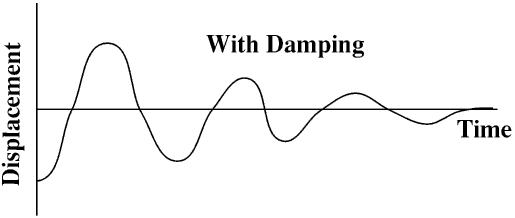© Barry Gray May 2003